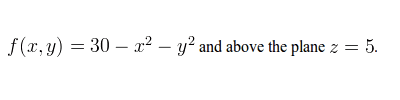# Setting up Double Intergrals to Calculate Volume

## Homework Statement

Set up the double integral in rectangular coordinates for calculating the volume.
The solid is created by the equation## Homework Equations

Fubini's Thereom ∫R∫f(x,y)dA = ∫ab∫f(x,y)dydx

## The Attempt at a Solution

The shape created by Z = 30 - x2 - y2 is 3d parabola facing downards.
Since it is bounded by Z = 5, the base shape is a circle at origin with radius 5 since
5 = 30 - x2 - y2 is equal to x2 + y2 = 25
As a result x is bounded by -5 and 5 while y is bounded by -√(25-x^2) and √(25-x^2)

As a result to set up the double integral
-55√(25-x^2)√(25-x^2)30-x^2-y^2dydx

Are the integral boundaries in correct?

Last edited:

## Homework Statement

Set up the double integral in rectangular coordinates for calculating the volume.
The solid is created by the equation## Homework Equations

Fubini's Thereom ∫R∫f(x,y)dA = ∫ab∫f(x,y)dydx

## The Attempt at a Solution

The shape created by Z = 30 - x2 - y2 is 3d parabola facing downards.
Since it is bounded by Z = 5, the base shape is a circle at origin with radius 5 since
5 = 30 - x2 - y2 is equal to x2 + y2 = 25
As a result x is bounded by -5 and 5 while y is bounded by -√(25-x^2) and √(25-x^2)

As a result to set up the double integral
-55-√(25-x^2)√(25-x^2)30-x^2-y^2dydx

Are the integral boundaries in correct?

Yes the boundaries are correct (except for the typo where you left out the "-" in the lower limit).

However, you really should be making use of the cylindrical symmetry here and changing to cylindrical coordinates. It makes the problem much easier.

Last edited:
Thanks for the response! However, that is the answer I entered into Webwork but it states it is incorrect.

Last edited:
Okay I managed to figure it out after reading: http://mathinsight.org/double_integral_volume

My boundaries were correct but the equation I was integrating was not.
It explained that the integral equation is not f(x,y) but f(x,y) - g(x,y), in my case g(x,y) being the "other surface" or z = 5

As a result I subtracted 5 from f(x,y) which was 30 - x^2 - y^2 and resulted in a final answer of
-55-√(25-x^2)√(25-x^2) 25-x^2-y^2dydx

Yes, what you had was the volume between the surface $z= 30- x^2- y^2$ and the xy-plane, z= 0. Unfortunately, so many basic problem ask for just the "area below the curve" and "the volume below the surface", we may develop the very bad habit of automatically assuming a lower bound of 0.
$$\int_{\theta= 0}^{2\pi}\int_{r= 0}^5 (30- r^2- 5) rdrd\theta=\int_{\theta= 0}^{2\pi}\int_{r= 0}^5 (25- r^2) rdrd\theta$$